×#### Thank you for registering.

One of our academic counsellors will contact you within 1 working day.

Click to Chat

1800-1023-196

+91-120-4616500

CART 0

• 0

MY CART (5)

Use Coupon: CART20 and get 20% off on all online Study Material

ITEM
DETAILS
MRP
DISCOUNT
FINAL PRICE
Total Price: Rs.

There are no items in this cart.
Continue Shopping• Complete JEE Main/Advanced Course and Test Series
• OFFERED PRICE: Rs. 15,900
• View Details

```Revision Notes on Gravitation

What is the Centripetal Force?

We know that an object in circular motion keeps on changing its direction.

Due to this, the velocity of the object also changes.

A force called Centripetal Force acts upon the object that keeps it moving in a circular path.

The centripetal force is exerted from the centre of the path.

Without the Centripetal Force objects cannot move in circular paths, they would always travel straight.

For Example, The rotation of Moon around the Earth is possible because of the centripetal force exerted by Earth.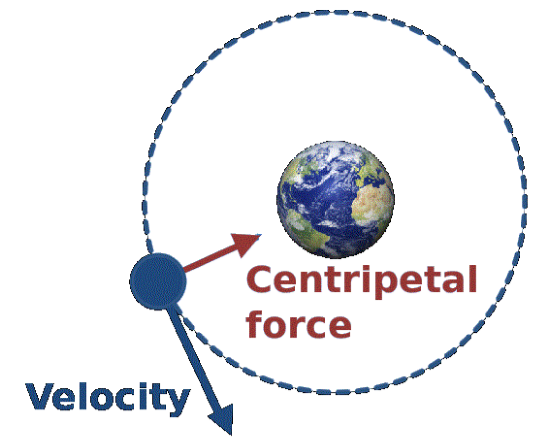Figure 1 Centripetal Force of Earth on Moon

Newton's Observations

Why does Apple fall on Earth from a tree? – Because the earth attracts it towards itself.

Can Apple attract the earth?  - Yes. It also attracts the earth as per Newton's third law (every action has an equal and opposite reaction). But the mass of the earth is much larger than Apple's mass thus the force applied by Apple appears negligible and Earth never moves towards it.

Newton thus suggested that all objects in this universe attract each other. This force of attraction is called Gravitational Force.Figure 2 Gravitational Force of Earth

Universal Law of Gravitation by Newton

According to the universal law of gravitation, every object attracts every other object with a force.

This force is directly proportional to the product of their masses.

This force is inversely proportional to the square of distances between them.

Consider the figure given below. It depicts the force of attraction between two objects with masses m1 and m2 respectively that are ‘d’ distance apart.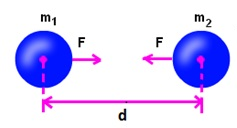The figure below describes how the universal law of gravitation is derived mathematically.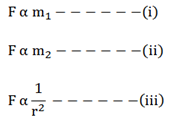From the above equation we can rewrite them as the following: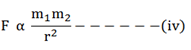If we remove the proportionality we get proportionality constant G as the following: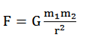The above equation is the mathematical representation of Newton’s universal Law of gravitation

Hence, G = Fr2/ m1 m2

SI Unit: Nm2 kg-2

Value of G = 6.673 × 10-11 Nm2 kg-2 (was found out by Henry Cavendish (1731- 1810))

The proportionality constant G is also known as the Universal Gravitational Constant​

Why we study the universal law of gravitation?

It explains many important phenomena of the universe –

Earth’s gravitational force

Why the moon always moves in a circular motion around the earth and the sun

Why all planets revolve around the sun

How the sun and moon can cause tides

Free Fall

Acceleration due to gravity – Whenever an object falls towards the Earth there is an acceleration associated with the movement of the object. This acceleration is called acceleration due to gravity.

Denoted by: g

SI Unit: m s-2

We know that, F= ma

Therefore, F = mg

The following figure demonstrates the mathematical derivation of ‘g'

The force (F) of gravitational attraction on a body of mass m due to earth of mass M and radius R is given byWe know from Newton’s second law of motion that the force is the product of mass and acceleration.

∴  F = ma

But the acceleration due to gravity is represented by the symbol g. Therefore, we can write

F = mg               ….. (2)

From the equation (1) and (2), we get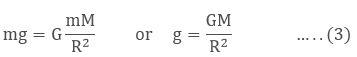When body is at a distance ‘r’ from the centre of the earth then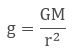Value of ‘g' may vary at different parts of the earth –

From the equation g = GM/ r2 it is clear that the value of ‘g' depends upon the distance of the object from the earth's centre.

This is because the shape of the earth is not a perfect sphere. It is rather flattened at poles and bulged out at the equator.

Hence, the value of ‘g' is greater at the poles and lesser at the equator. However, for our convenience, we take a constant value of ‘g' throughout.

We can find the value of acceleration due to gravity by the following –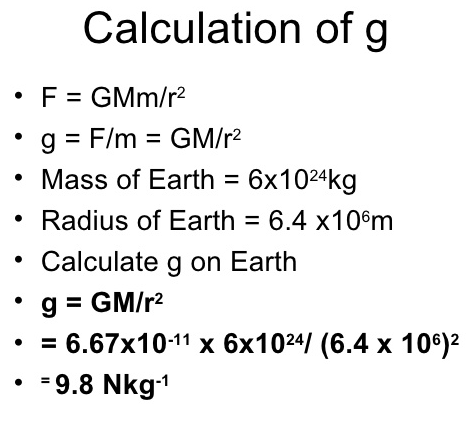What is Free Fall?

When an object falls towards the earth due to earth’s gravity and no other force is acting upon it, the object is said to be in free fall state. Free falling objects are not even resisted by the air.

g = 9.8 m/s2 is also called the Free-fall Acceleration.

Value of ‘g' is same on the earth, so the equations of motion for an object with uniform motion are valid where acceleration ‘a' is replaced by ‘g', as given under:

v = u + gt

s = ut + (1/2) gt2

2 g s = v2 – u2

Consider the equations of motion given in different scenarios:

When an object at rest falls towards earth – its initial velocity is zero

v = gt

s = t + (1/2) gt2

2 g s = v2

When an object with some initial velocity (u) falls towards earth –

v = u + gt

s = ut + (1/2) gt2

2 g s = v2 – u2

When an object is thrown upwards from earth – the gravitational force acts in opposite direction, hence g is negative

v = u - gt

s = ut - (1/2) gt2

-2 g s = v2 – u2

Difference between Universal gravitational Constant and Acceleration due to Gravity

Mass
Weight

Mass is defined as the quantity of matter in an object.

The weight of an object is the force by which the gravitational pull of earth attracts the object.

Mass is a scalar quantity

Weight is a vector quantity

The mass of an object is always constant as it depends upon the inertia of the object

The weight of an object can vary at different locations because of change in gravitational force of the earth

Mass can never be zero

Weight can be zero at places there is no gravitational force

Denoted as: m

Denoted  as W

F = mg

where m = mass of object

a = acceleration due to gravity

Similarly, W is force, so

W = mg

SI Unit: kg

SI unit: N

Weight of an object on the Moon

Just like the Earth, the Moon also exerts a force upon objects. Hence, objects on moon also have some weight. The weight will not be same as than on the earth. So, weight on the Moon can be calculated as -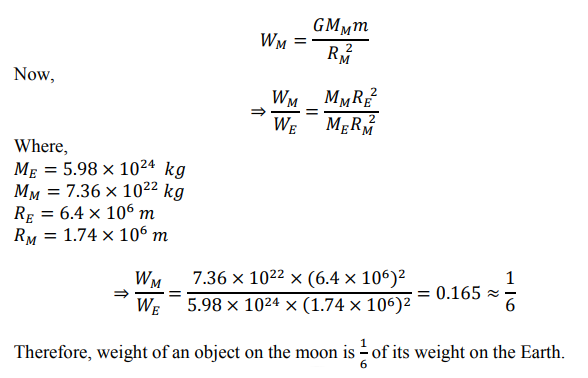Thrust and Pressure

Thrust

The force that acts in the perpendicular direction is called thrust.

It is similar to force applied to an object

It is a vector quantity.

Pressure

The force that acts per unit area of the object is pressure.

It is the thrust per unit area.

Pressure is denoted by ‘P'

P = thrust/ area = force/ area = F/A

SI unit: N/m2 or Pa (Pascal)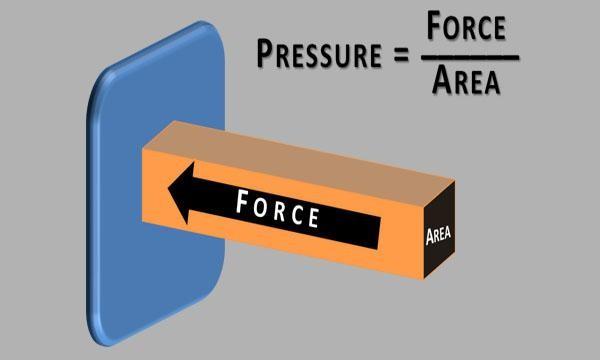Figure 4 Pressure

Why do nails have sharp edges?

We know that pressure is inversely proportional to area. As area increases, pressure decreases and vice versa. So, nails' sharp edges make it easier for them to get into the wall because more pressure is exerted on the wall from a single point.

Solids - They exert pressure on the surface because of their weight.

Fluids (gases and liquids) - They also have weight, therefore, they exert pressure on the surface and the walls of the container in which they are put in.

Buoyancy

Whenever an object is immersed in a liquid, the liquid exerts a buoyant force or upthrust in the opposite direction of the gravitational force. This is also called the Force of Buoyancy.

It depends upon the density of the fluid.

Therefore an object is able to float in water when the gravitational force is less than the buoyant force.

Similarly, an object sinks into the water when the gravitational force is larger than the buoyant force.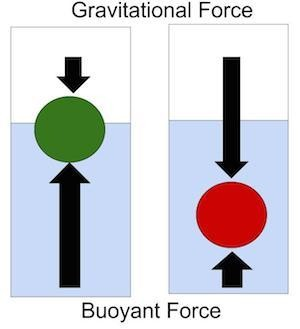Figure 5 Buoyancy

Why does an object sink or float on water?

An object can sink or float on water based on its density with respect to water. The density is defined as mass per unit volume.

Objects having a density less than water float in it. For Example, Cork flows in water because its density is lower than that of water.

Objects that have a density higher than water sink in it. For Example, Iron nail sinks in water because the density of iron is more than water's density.

Thus, we can conclude that buoyancy depends upon:

The density of the liquid

The volume of the object (as the volume of object increases, its density decreases and vice-versa)

Archimedes Principle

According to the Archimedes principle, whenever an object is immersed in a liquid (fully or partially), the liquid exerts an upward force upon the object. The amount of that force is equivalent to the weight of the liquid displaced by the object.

This means that if the weight of an object is greater than the amount of liquid it displaces, the object will sink into the liquid. However, if the weight of an object is less than the amount of water it displaces, the object will sink.

Submarines have a tank called Buoyancy Tank. Whenever the submarine needs to be taken inside water the tank is filled which thus increases the weight of the submarine. Similarly, when the submarine is to appear above water the tank is emptied and the weight of the submarine becomes lighter and it rises above the water.

Ships are heavier than water but their unique shape gives them a large volume. Their volume is larger than their weight and hence the water displaced by a ship provides it with the right upthrust so that it can float on water.

Applications of Archimedes Principle

In evaluating relative density

In designing ships and submarines

In making lactometers and hydrometers

What is relative density?

When density can be expressed in comparison with water's density it is called Relative Density. It has no unit because it is a ratio of two similar quantities.Why water is chosen as a reference?

Water is present everywhere on earth so it becomes easier to evaluate the density of a substance in relation to water.

How relative density can be used as a measure to determine in an object will sink or float in water?

Relative Density of an object
Float / Sink

Greater than 1
Sink in water

Less than 1
Float in water

```### Course Features

• 728 Video Lectures
• Revision Notes
• Previous Year Papers
• Mind Map
• Study Planner
• NCERT Solutions
• Discussion Forum
• Test paper with Video Solution